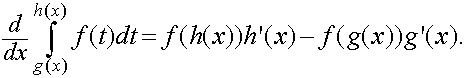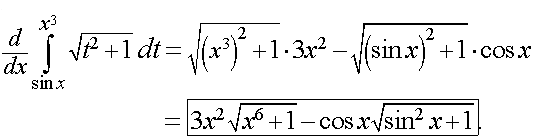# what is newton -leibnitz's rule how to apply it in a question ?explain with 3-4 exampleBadiuddin askIITians.ismu Expert
148 Points
12 years ago

Leibniz Integral Rule

The Leibniz integral rule gives a formula for differentiation of a definite integral whose limits are functions of the differential variable,where the partial derivative of f indicates that inside the integral only the variation of ƒ ( x, α ) with α is considered in taking the derivative.

### Example

Here, we consider the integration of$\textbf I\;=\;\int_0^{\frac{\pi}{2}}\,\frac{1}{\left(a\,\cos^2\,x+b\,\sin^2\,x\right)^2}\;dx,\,$

where both$a,\,b\,>\,0$, by differentiating under the integral sign.

Let us first find$\textbf J\;=\;\int_0^{\frac{\pi}{2}}\,\frac{1}{a\,\cos^2\,x+b\,\sin^2\,x}\;dx.\,$

Dividing both the numerator and the denominator by$\cos^2\,x$ yields\begin{align} \textbf J\; &=\;\int_0^{\frac{\pi}{2}}\,\frac{\sec^2\,x}{a\,+b\,\tan^2\,x}\;dx \\ &=\,\frac{1}{b}\,\int_0^{\frac{\pi}{2}}\,\frac{1}{\left(\sqrt{\,\frac{a}{b}\,}\right)^2+\tan^2\,x}\;d(\tan\,x)\, \\ &=\,\frac{1}{\sqrt{\,a\,b\,}}\,\left(\tan^{-1}\left(\sqrt{\,\frac{b}{a}\,}\,\tan\,x\right)\right)\,\bigg|_0^{\frac{\pi}{2}}\;=\;\frac{\pi}{2\,\sqrt{\,a\,b\,}}. \end{align}

The limits of integration being independent of$a,\,$$\textbf J\;=\;\int_0^{\frac{\pi}{2}}\,\frac{1}{a\,\cos^2\,x+b\,\sin^2\,x}\;dx\,$ gives us$\frac{\partial\,\textbf J}{\partial\,a}\;=\;-\,\int_0^{\frac{\pi}{2}}\,\frac{\cos^2\,x\;dx}{\left(a\,\cos^2\,x+b\,\sin^2\,x\right)^2}\,$

whereas$\textbf J\;=\;\frac{\pi}{2\,\sqrt{\,a\,b\,}}$ gives us$\frac{\partial\,\textbf J}{\partial\,a}\;=\;-\frac{\pi}{4\,\sqrt{\,a^3\,b\,}}.\,$

Equating these two relations then yields$\,\int_0^{\frac{\pi}{2}}\,\frac{\cos^2\,x\;dx}{\left(a\,\cos^2\,x+b\,\sin^2\,x\right)^2}\;=\;\frac{\pi}{4\,\sqrt{\,a^3\,b\,}}.\,$

In a similar fashion, pursuing$\frac{\partial\,\textbf J}{\partial\,b}\,$ yields$\,\int_0^{\frac{\pi}{2}}\,\frac{\sin^2\,x\;dx}{\left(a\,\cos^2\,x+b\,\sin^2\,x\right)^2}\;=\;\frac{\pi}{4\,\sqrt{\,a\,b^3\,}}.\,$

Adding the two results then produces$\textbf I\;=\;\int_0^{\frac{\pi}{2}}\,\frac{1}{\left(a\,\cos^2\,x+b\,\sin^2\,x\right)^2}\;dx\;=\;\frac{\pi}{4\,\sqrt{\,a\,b\,}}\left(\frac{1}{a}+\frac{1}{b}\right),\,$

which is the value of the integral$\textbf I.\,$

Please feel free to post as many doubts on our discussion forum as you can.
If you find any question Difficult to understand - post it here and we will get you the answer and detailed solution very quickly.

All the best.

Regards,Kushagra Madhukar
2 years ago
Dear student,

The Newton – Leibnitz’s law can be stated as followsExample-Thanks and regards,
Kushagra